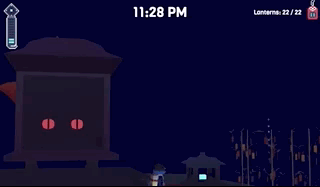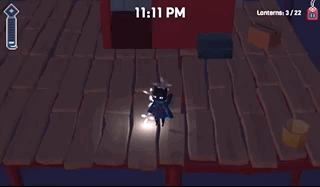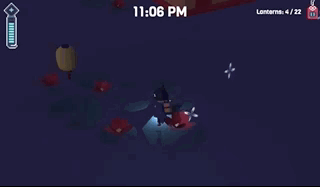# Summary

Particle systems are an awesome way to add more depth to actions & movements in a game. For any particle system you're thinking about creating, test it in a playground first. The playground has a particle editor that makes everything a lot easier! I tested out all of my particle systems in the playground before bringing them into the game and figuring positioning and making minor adjustments. It's also really useful if you're going to end up duplicating the same particle system several times as all you need to do is perfect 1.

# Fireworks

The most important particle system of my game was the fireworks as it was the only one that was essential to the game. This was supposed to give an awesome ending! I used a playground as reference on how to make the fireworks. You can't actually use the exact code that's here in a local project because it uses some properties of the particle system that are only available in the playground. So, I had to make some adjustments to the code to get it to work.

Here's the original Playground -

Here's the modified Playground -## Setting Up the Particle Systems

Similar to this playground, I made a Firework class. There are two parts to a firework:

1. The Rocket
The rocket is a particle system created in the constructor of the Firework. We start off by creating an emitter for the rocket. This is the mesh that's going to have the particle system attached to it so that the particle system moves along with its position.
``````const sphere = Mesh.CreateSphere("rocket", 4, 1, scene);
sphere.isVisible = false;
//the origin spawn point for all fireworks is determined by a TransformNode called "fireworks", this was placed in blender
let randPos = Math.random() * 10;
sphere.position = (new Vector3(scene.getTransformNodeByName("fireworks").getAbsolutePosition().x + randPos * -1, scene.getTransformNodeByName("fireworks").getAbsolutePosition().y, scene.getTransformNodeByName("fireworks").getAbsolutePosition().z));
this._emitter = sphere;
//set how high the rocket will travel before exploding and how long it'll take before shooting the rocket
this._height = sphere.position.y + Math.random() * (15 + 4) + 4;
this._delay = (Math.random() * i + 1) * 60; //frame based
``````
We need to determine a few things for our Firework:
1. Position
• The position of the rocket is random only across the x-axis. This ensures that all of the fireworks fire the same distance away, but along a line.
2. Height
• The height is random between 4 and 15 just to give some variety to the fireworks.
3. Start Time
• I wanted the fireworks to stagger so that they all didn't go off at once. This extended the duration of the entire fireworks show as well without having to create a ton. _delay is a number between 1 and the index of the firework. Multiply this by 60 and we have how many frames it will wait before shooting the rocket.
``````//Rocket particle system
let rocket = new ParticleSystem("rocket", 350, scene);
rocket.particleTexture = new Texture("./textures/flare.png", scene);
rocket.emitter = sphere;
rocket.emitRate = 20;
rocket.minEmitBox = new Vector3(0, 0, 0);
rocket.maxEmitBox = new Vector3(0, 0, 0);
rocket.color1 = new Color4(0.49, 0.57, 0.76);
rocket.color2 = new Color4(0.29, 0.29, 0.66);
rocket.colorDead = new Color4(0, 0, 0.2, 0.5);
rocket.minSize = 1;
rocket.maxSize = 1;
this._rocket = rocket;
``````
The particle system itself just uses a simple texture and decreases in size over its lifetime.
2. The Explosion
The explosion has its own function _explosions that creates another particle system. It's a bit more involved, but I'll do my best to try and explain what is going on:
``````const explosion = Mesh.CreateSphere("explosion", 4, 1, this._scene);
explosion.isVisible = false;
explosion.position = position;
let emitter = explosion;
//grabbing vertex data
emitter.useVertexColors = true;
let vertPos = emitter.getVerticesData(VertexBuffer.PositionKind);
let vertNorms = emitter.getVerticesData(VertexBuffer.NormalKind);
let vertColors = [];
``````
First, we create a mesh that's going to be the shape of our explosion, a sphere. We're going to be using the vertices of this mesh to create particle systems. In order to do that, we need the vertex data of this mesh.
``````for (let i = 0; i < vertPos.length; i += 3) {
let vertPosition = new Vector3(
vertPos[i], vertPos[i + 1], vertPos[i + 2]
)
let vertNormal = new Vector3(
vertNorms[i], vertNorms[i + 1], vertNorms[i + 2]
)
let r = Math.random();
let g = Math.random();
let b = Math.random();
let alpha = 1.0;
let color = new Color4(r, g, b, alpha);
vertColors.push(r);
vertColors.push(g);
vertColors.push(b);
vertColors.push(alpha);
//..emitter for the particle system
//..actual particle system for each exploding piece
}
emitter.setVerticesData(VertexBuffer.ColorKind, vertColors);
``````
For every third vertex position, we store a new position and normal as vector3s. We then generate a random color for this vertex. After we've set up our new particle system based off of vertex data, we need to actually update the emitter to use the colors we generated.
``````//emitter for the particle system
let gizmo = Mesh.CreateBox("gizmo", 0.001, this._scene);
gizmo.position = vertPosition;
gizmo.parent = emitter;
let direction = vertNormal.normalize().scale(1); // move in the direction of the normal
``````
Still in this for loop, we create an emitter mesh, similar to how we did for the rocket except this time, the emitter is positioned at the vertPosition that we created. It's also been given a direction to move based off of the vertNormal. This will cause it to move straight out (like an explosion).
``````//actual particle system for each exploding piece
const particleSys = new ParticleSystem("particles", 500, this._scene);
particleSys.particleTexture = new Texture("textures/flare.png", this._scene);
particleSys.emitter = gizmo;
particleSys.minEmitBox = new Vector3(1, 0, 0);
particleSys.maxEmitBox = new Vector3(1, 0, 0);
particleSys.minSize = .1;
particleSys.maxSize = .1;
particleSys.color1 = color;
particleSys.color2 = color;
particleSys.colorDead = new Color4(0, 0, 0, 0.0);
particleSys.emitRate = 500;
particleSys.gravity = new Vector3(0, -9.8, 0);
particleSys.direction1 = direction;
particleSys.direction2 = direction;
particleSys.minEmitPower = 10;
particleSys.maxEmitPower = 13;
particleSys.targetStopDuration = 0.2;
particleSys.disposeOnStop = true;
particleSys.start(); //automatically start once created
``````
The last step is to make the actual particle system.

## Firing the Particle Systems

Now we have a particle system set up for a firework, but we want to have multiple. In addition, we need to figure out when to trigger the fireworks to start. The explosions start automatically once they're created, but what about the rocket?

### Create Instances

Before we can use the particle systems, we need to create instances of the Firework class. In the Environment constructor, I just looped through how many fireworks I wanted and then added them to an array of fireworks.

``````//--FIREWORKS--
for (let i = 0; i < 20; i++) {
this._fireworkObjs.push(new Firework(this._scene, i));
}
``````

Then, add an observable that checks to see if the fireworks have started.

``````this._scene.onBeforeRenderObservable.add(() => {
this._fireworkObjs.forEach(f => {
if (this._startFireworks) {
f._startFirework();
}
})
})
``````

### Starting Fireworks

Once we are in the "win state", we trigger the fireworks to start by setting _startFireworks to true. Now, _startFirework should be called for each firework in the environment:

``````if(this._started) { //if it's started, rocket flies up to height & then explodes
if (this._emitter.position.y >= this._height && !this._exploded) {
//transition to the explosion particle system
this._exploded = !this._exploded; // don't allow for it to explode again
this._explosions(this._emitter.position);
this._emitter.dispose();
this._rocket.stop();
} else {
//move the rocket up
this._emitter.position.y += .2;
}
} else {
//use its delay to know when to shoot the firework
if(this._delay <= 0){
this._started = true;
//start particle system
this._rocket.start();
} else {
this._delay--;
}
}
``````

Once the delay has reached 0, the rocket will shoot. After the rocket reaches the maximum height, it will explode and the emitter will be disposed of.

# Player Sparkler

Creating the player sparkler's particle system made it feel more like a sparkler and brought emphasis to it. Before, you could really only see that there was light by the player but it didn't really stand out as a sparkler.

I made a function _createSparkles that made the particle system. This is called in the Player constructor (characterController.ts).

In order to toggle this on/off when the sparkler goes out, I just passed the object into startSparklerTimer and stopSparklerTimer then called sparkler.start() and sparkler.stop() respectively.You can look at how I made the particle system in this Playground Demo -

# Lantern Stars

I wanted to give some sort of extra feedback to the player when they lit a lantern so that it felt like an accomplishment. I decided to add this really simple star particle system.I created a _loadStars function that made the particle system and called it in the Lantern constructor. Then, when setEmissiveTexture is called, I started the particle system.

You can look at how I made the particle system in this Playground Demo -

It took a bit of messing around with to get the exact effect, so this is where the editor can come in super handy as you can just make quick edits to your particle systems. Just remember that if you want there to be a set duration, assign that last as the particle system won't loop in the editor if you have this set.